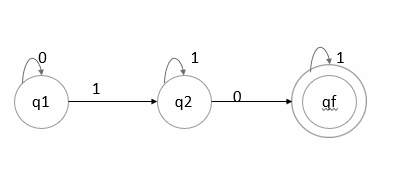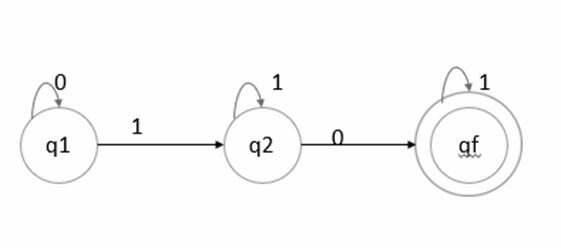# What is finite automata?

Finite automata is an abstract computing device. It is a mathematical model of a system with discrete inputs, outputs, states and a set of transitions from state to state that occurs on input symbols from the alphabet Σ.

## Finite Automata Representation

The finite automata can be represented in three ways, as given below −

• Graphical (Transition diagram)
• Tabular (Transition table)
• Mathematical (Transition function)

## Formal definition of Finite Automata

Finite automata is defined as a 5-tuples

M=(Q, Σ, δ,q0,F)

Where,

• Q: Finite set called states.
• Σ: Finite set called alphabets.
• δ: Q × Σ → Q is the transition function.
• q0 ∈ Q is the start or initial state.
• F: Final or accept state.

Finite Automata can be represented as follows −

• Transition diagram
• Transition table
• Transition function

## Transition Diagram

It is a directed graph associated with the vertices of the graph corresponding to the state of finite automata.

An example of transition diagram is given below −Here,

• {0,1}: Inputs
• q1: Initial state
• q2: Intermediate state
• qf: Final state

## Transition table

It is basically a tabular representation of the transition function that takes two arguments (a state & a symbol) and returns a value (the ‘next state’).

δ : Q × Σ → Q

In transition table, the following factors are considered −

• Rows correspond to state.
• Column corresponds to the input symbol.
• Entries correspond to the next state.
• The start state is marked with ->.
• The accept state marked with *.

An example of transition table is as follows −The transition table is as follows −

State/input symbol01
->q1q1q2
q2qfq2
qf-qf

## Transition function

The transition function is denoted by δ. The two parameters mentioned below are the passes to this transition function.

• Current state
• Input symbol

The transition function returns a state which can be called as the next state.

δ (current_state, current_input_symbol) = next_state

For example, δ(q0,a)=q1